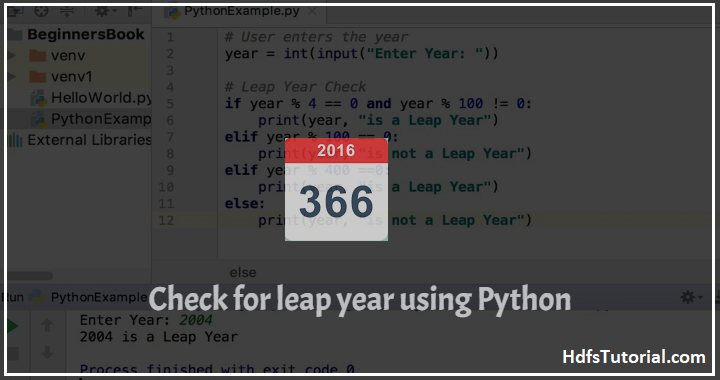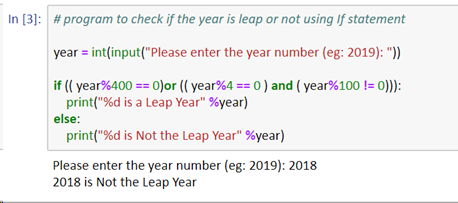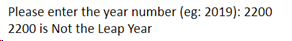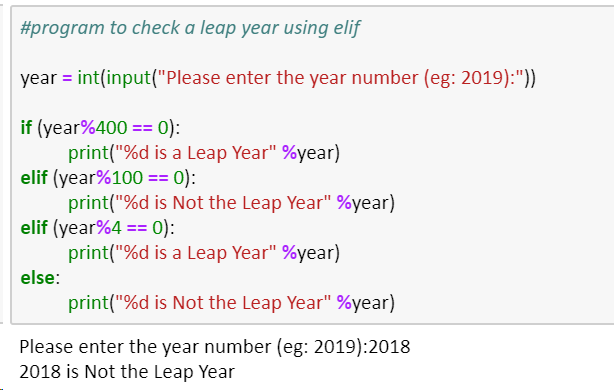# Leap Year Program In Python- 2 Methods

In this post, we’re going to talk about how to check leap year in python. This leap year program in python will help you check any year and return if that is a leap year or not.

But before going into the leap year program in python, let’s understand what is leap year.

##What is leap year

Leap year is that year in which we have 366 days. Usually we have 365 days in a year. And so, if you need to identify whether the year is leap year or not, simply divide the year with 4 and if the remainder is 0 then it is a leap year.

But there is one twist!

For century years like 2000, 2500, 1200 etc. we need to check further to decide whether it is a leap year or not. For such century years, we need to do the following-

• If the year is completely divided by 400 then that year is a leap year
• Else it won’t be a leap year

Now let’s start and convert the same logic in python and build a program to check leap year in python.

## leap year program in python using If statement

If statements are the easiest conditional statement and so let’s start and see how to identify a leap year in python using If statement.

```# program to check if the year is leap or not using If statement

year = int(input("Please enter the year number (eg: 2019): "))

if (( year%400 == 0)or (( year%4 == 0 ) and ( year%100 != 0))):
print("%d is a Leap Year" %year)
else:
print("%d is Not the Leap Year" %year)```Now let’s see what we are doing here:

• First, we checked if the year is divisible by 400 or 4 and then not divisible by 100. This way we can correctly say it is leap year.
• This is because if the year is divisible by 4 and 100 both then it won’t be leap. Year 2200 is an example of it.

So, either of divisible by 4 or 400 should be correct and must not be completely divisible by 100.

## Python leap year program using elif statement

Here we are going to use the elif statement in python to check if the given year is a leap year or not. Just we are expanding the above code and adding else statement as shown below-

```#program to check a leap year using elif

year = int(input("Please enter the year number (eg: 2019):"))

if (year%400 == 0):
print("%d is a Leap Year" %year)
elif (year%100 == 0):
print("%d is Not the Leap Year" %year)
elif (year%4 == 0):
print("%d is a Leap Year" %year)
else:
print("%d is Not the Leap Year" %year)```Here we have done the following:

• First we have asked the user to provide the year number
• Then we have checked if the number is divisible by 400 or not. As per the algo, if any number if divisible by 400 is a leap year. If not then the control will pass to elif statement.
• Now if any number is not completely divisible by 400 and divisible by 100. If this is true then it won’t be a leap year. If this fails, then the 3rd statement will be executed. That statement will be executed if and only if the above two fails. Means if after dividing the number by 400, remainder is not 0 and also after dividing by 100, reminder is not 0.
• Then it will check if it is completely divisible by 4 or not. If it is, then it is a leap year else no.

This way, you can easily identify if a given year is a leap year or not. There can be other ways also in which you can write leap year program in python which you may explore further.

### Conclusion-

This was all about leap year program in python. We saw two methods to write a leap year program in python. You may use any of the above and try.

Please comment if you’ll face any challenge. For such more Python code snippets, you can check our python section.

##### Leap year program in python
5

Summary

Follow these 2 methods to check if the year given is a leap year or not. You can simply check using either of the methods and follow.